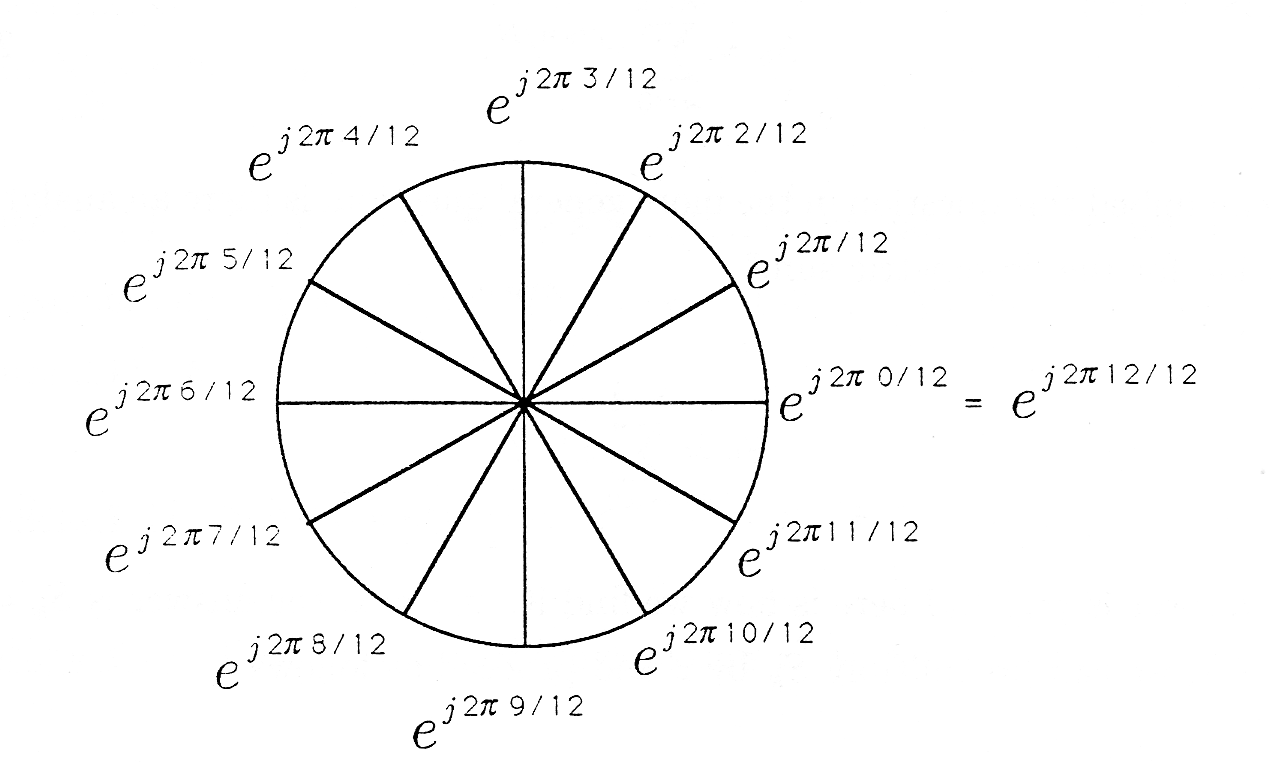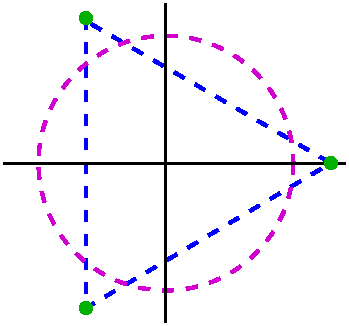×#### Thank you for registering.

One of our academic counsellors will contact you within 1 working day.

Click to Chat

1800-1023-196

+91-120-4616500

CART 0

• 0

MY CART (5)

Use Coupon: CART20 and get 20% off on all online Study Material

ITEM
DETAILS
MRP
DISCOUNT
FINAL PRICE
Total Price: Rs.

There are no items in this cart.
Continue Shopping```nth Roots of UnityIn general, the term root of unity which is also termed as de Moivre number is basically a complex number which, when raised to some integer n gives the result as 1.

Mathematically, if n is a positive integer, then ‘x’ is said to be an nth root of unity if it satisfies the equation xn = 1. Thus, this equation has n roots which are also termed as the nth roots of unity.

How do We Find the nth Root of Unity?

As stated above, if x is an nth root of unity, then it satisfies the relation xn = 1.

Now, ‘1’ can also be written as cos 0 + i sin 0.

So, we have, xn = 1

= cos 0 + i sin 0

= cos 2kπ + i sin 2kπ   [k is an integer]

Taking the nth root on both sides, we get

x = (cos 2kπ + i sin 2kπ)1/n
= (cos (2kπ/n) + i sin (2kπ/n)) where k = 0 , 1, 2 , 3 , 4 , ……… , (n-1)

So each root of unity is

x = cos [(2kπ)/n] + i sin[(2kπ)/n] where 0 ≤ k ≤ n-1.

The complex numbers are in the form x+iy and are plotted on the argand or the complex plane. Also, since the roots of unity are in the form cos [(2kπ)/n] + i sin[(2kπ)/n], so comparing it with the general form of complex number, we obtain the real and imaginary parts as

x = cos[(2kπ)/n] ,  y = sin[(2kπ)/n].
Now, we see that the values of x and y satisfy the equation of unit circle with centre (0,0) i.e. x2 + y2 = 1.Now, we consider some of the key results on nth roots of unity-

The cube roots of unity are 1, (-1+i√3)/2, (-1-i√3)/2.
If w is one of the imaginary cube root of unity then 1+ w + w2 = 0. In general 1 + wn + w2n = 0; where n ∈ I but is not the multiple of 3.
In polar form the cube roots of unity are:

cos 0 + i sin 0; cos 2π/3 + isin 2π/3; cos 4π/3 + I sin 4π/3.

Product of the nth roots of any complex number z is z(-1)n-1

The three cube roots of unity when plotted on the argand plane constitute the vertices of an equilateral triangle.The following factorization should be remembered:

(a, b, c ∈ R and w is the cube root of unity)

a3 - b3= (a-b) (a-wb) (a-x2b);

x2 +x+1=(x-w)(x-w2);

a3 + b3= (a+b) (a+wb) (a+x2b);

a2 +ab+b2 = (a-bw)(a-bw2)

a3 + b3 + c3 - 3abc = (a+b+c) (a+wb+w2c) (a+w2b+wc);

View the video for more on nth roots of unity

nth Roots of unity -

If 1, α1,  α2,......αn-1 are the n, nth roots of unity then:They are in G.P. with common ratio i(2π/n)
1p + α1p + α2p + .... αn-1p = 0 if p is not an integral multiple of n.
(1 - α1)(1 - α2).......(1 - αn-1) = n and,
(1 + α1)(1 + α2).......(1 + αn-1) = 0 if n is even and

= 1 if n is odd.

α1 . α2 . α3 ...... αn-1 = 1 or -1 according as n is odd or even.

The sum of the following series should be remembered:

(i) cos θ + cos 2θ + cos 3θ + ..................... + cos nθ

= (sin (nθ/2)/sinθ/2) cos((n+1)/2)θ

(ii) sin θ + sin 2θ + ..................... + sin nθ

= (sin(nθ/2)/sinθ/2) sin((n+1)/2)θ

Remark: If θ = 2π/2 then the sum of the above series vanishes.

Logarithm of a complex quantity -

(i) Loge(α + iβ) = 1/2 loge(α2 + β2) + i(2nπ + tan-1(β/α))  where n ∈ I.

(ii) ii represents a set of positive real numbers given by e-(2nπ + π/2), n ∈ I.

Illustration: Find the smallest positive integer n for which [(1+i)/ (1-i)]n  = 1. (1980)

Solution: The given expression is [(1+i)/ (1-i)]n = 1

First, we rationalize the given expression by multiplying and dividing the given expression by the conjugate of denominator,

[(1+i)/(1-i) . (1+i)/(1+i)]n = 1

This means (2i/2)n = 1

This gives in =1.

The smallest positive integer n for which in =1 is 4.

Hence, the value of n is 4.

Illustration: Let z1 and z2 be nth roots of unity which subtend a right angle at the origin, then what should be the form of n if k is an integer?

Solution: We know that by the formula of argument,

arg z1/ z2 = π/2

Hence, z1/ z2 = cos π/2 + isin π/2 = i

So, z1n/ z2n = (i)n = 1

Hence, n = 4k.

askIITians is a unique online portal which offers a platform where students are free to ask any kind of questions on topics like cube root of unity in complex numbers, imaginary cube root of unity or fourth root of unity.

Related Resources

Look into the Solved Papers of Previous Years to get a hint of the kinds of questions asked in the exam.

You can get the knowledge of Important Books of Mathematics

To read more, Buy study materials of Complex Numbers comprising study notes, revision notes, video lectures, previous year solved questions etc. Also browse for more study materials on Mathematics here.
```### Course Features

• 731 Video Lectures
• Revision Notes
• Previous Year Papers
• Mind Map
• Study Planner
• NCERT Solutions
• Discussion Forum
• Test paper with Video Solution